# "Distortion", the incorrect and correct usage of the word

Written by Paul Bourke
July 2020

The following is a response to the use of the word "distortion" in the context of image projections, notably fisheye, cylindrical panoramas and equirectangular projections. While there are negative effects related to practical image recording that can be referred to as a distortion, there are other effects of image projections that are inherent in the mathematics and as such calling them "distortions" is inappropriate.

In the discussion here, examples will be based upon the following equirectangular image.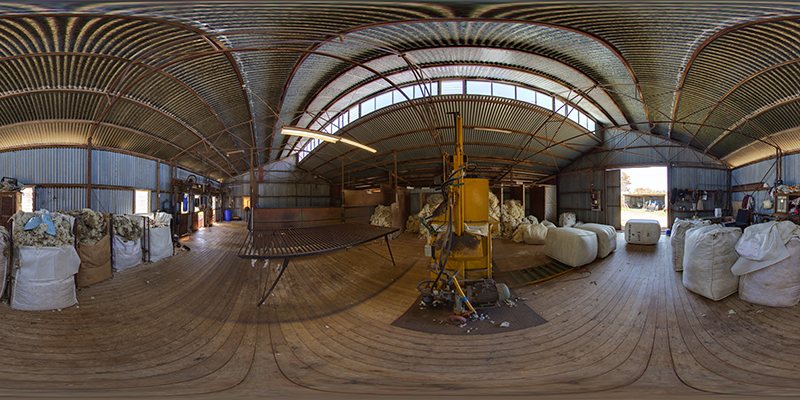Equirectangular projection

It is images such as this that are often referred to as distorted. This often arises because lines that one knows are straight in the real world do not appear straight in the image, like they do in a perspective image. One also notes that as one moves towards the north and south pole, objects get increasingly stretched horizontally. At the extreme top and bottom edge of the image a single point in the scene is stretched along the entire edge.

Similar curvature of straight lines can be observed in fisheye projections as seen in the following.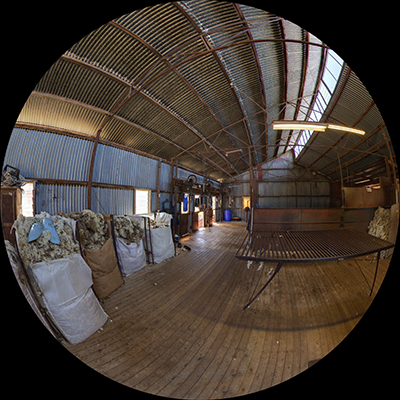180 degree fisheye projection

The word "distortion" carries with it negative connotations, of something not being correct or perfect. The basic argument as to why it is not an appropriate term when referring to the visual effects in these projections is that they are based on very precise mathematics. They are a very well defined way of projecting a 3D scene onto a 2D plane. The effects being referred to as distortions are in comparison to the more familiar perspective projection where indeed straight lines in the scene appear straight in the perspective projection. See later for visual effects that do arise in perspective views. It should be noted that the reason why projections such as fisheye and equirectangular are even used is because the same visual field of view cannot be represented in a single perspective projection.

The correct way to think about these projections is as mappings from 3D to 2D. Given the different topologies it is fundamentally impossible to project 3D to 2D without giving rise to these visual effects. Something has to be sacrificed and this is the domain of mapping, one introduces variation in area across the image, of angles, or scale. Once the mathematical transformation is known then any projection can be derived from any other. So for example, if one doesn't "like" the effect at the top of the above image one can simply change the mapping, this can be done in many ways. Examples provided below include reorientating the equirectangular, noting that while this may seem strange it encapsulates exactly the same visual information as the more standard orientation.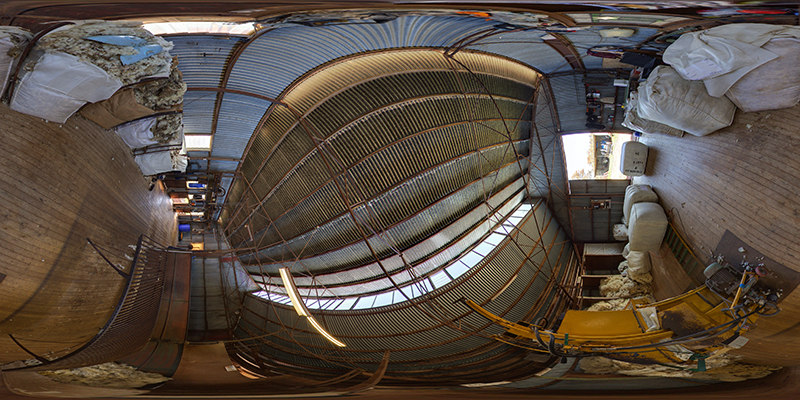Looking up, equirectangular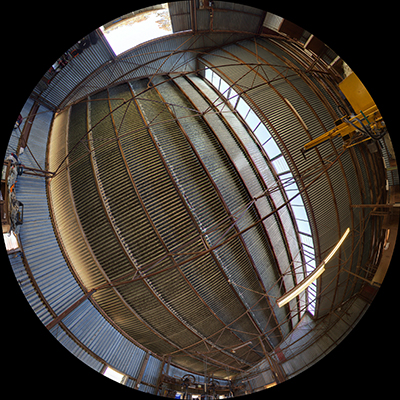Looking up, fisheyeLooking up, perspective

So, what can the word "distortion" be legitimately be used for. When capturing a 3D scene with a real camera and lens, there are effects that mean the resulting image deviates from a pure perspective projection. We are familiar with these and give them names such as pincushion and barrel distortion depending on whether parallel lines converge or diverge. This is the correct usage of the word distortion, something that deviates from the perfect. When using photographed image where the correct mathematical relationships are required, the first thing one normally does is correct for those distortions, turning the captured image into a perfect perspective, a pinhole camera model.Lens barrel distortionLens pincushion distortion

The same process occurs when capturing a 3D scene with a fisheye lens. There is well a well defined mathematical formulation for a linear fisheye projection but any physically realised fisheye optics is going to deviate from that, that is a distortion. As in the perspective situation, if one is going to use a photographically captured fisheye in a precise way (for example stitching two together to form an equirectangular) then inherent in that operation is a correction for the deviation of the captured fisheye from the ideal fisheye. This is discussed in more detail here: Fisheye lens correction.

A final word on the perception that perspective projections are the gold standard. While they too are precisely defined mathematical projections and so also any effects cannot be called distortion, they do exhibit visual effects. Consider a perspective projection of a scene containing a perfect sphere. The projection of the sphere might be expected to be a circle but that only occurs in one case, namely where the sphere is located exactly in the center of the camera. For all other compositions of the sphere in the image frame the appearance of the sphere will be oblong, not a circle at all. This can be seen by inspecting the center wool bale in the image on the left below, compared to the off-axis shape when it is not in the center of the camera view.Central wool baleOff center wool bale

The other visual effect, actually the same root cause as the above, is the whole reason why fisheye and equirectangular projections are used instead perspective. This is the effect that occurs as the field of view increases. In the left image below the perspective projection has a 60 degree horizontal field of view and the scene looks as expected. On the the right there is a 140 degree horizontal field of view, with the extreme stretching the further one looks away from the center of the view. This arises due to the tan() relationship between position on the image and angle into the scene, again not fair to call it a "distortion" because it is a natural consequence of the mathematics.Perspective 60 degrees FOV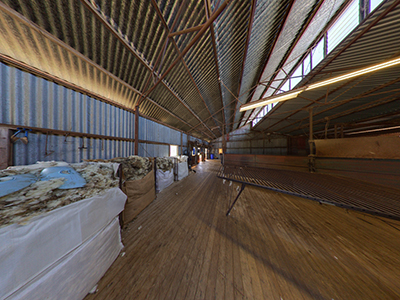Perspective 140 degrees FOV

Historically the word distortion in reference to map projections arose because one could not read distances, areas, angles etc directly from the 2D projection. In that sense the use of distortion is fair enough, but it is a concept we can dispense with given a digital map projection because we can measure any quantity correctly as long as we take into account the projection mathematics. We are no longer constrained to only reading quantities directly off the 2D image.